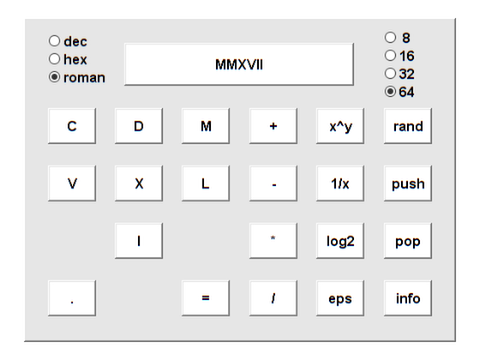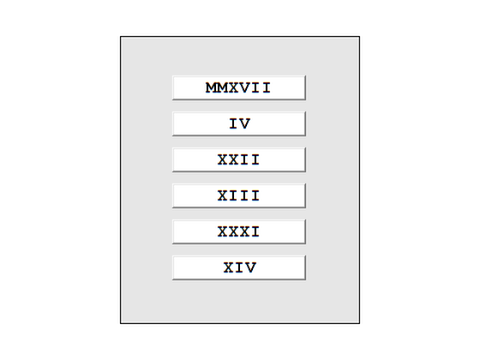# A Roman Numeral Object, with Arithmetic, Matrices and a Clock

A MATLAB object for arithmetic with Roman numerals provides an example of object oriented programming. I had originally intended this as my April Fools post, but I got fascinated and decided to make it the subject of a legitimate article.

### Contents

#### Background

I've always been interested in Roman numerals. In my former life as a professor, when I taught the beginning computer programming course, one of my projects involved Roman numerals.

Doing arithmetic with Roman numerals is tricky. What is IV + VI ? Stop reading this blog for a moment and compute this sum.

Did you find that IV + VI = X ? How did you do that? You probably converted IV and VI to decimal, did the addition using decimal arithmetic, then converted the result back to Roman. You computed 4 + 6 = 10. That is also how my roman object works. I have no idea how the Romans did it without decimal arithmetic to rely on.

#### Roman Numerals

Recall that Roman numerals are formed from seven letters

  I, V, X, L, C, D, and M.

Their values, expressed in decimal, are

  1, 5, 10, 50, 100, 500, and 1000.

A Roman numeral is just a string of these letters, usually in decreasing order, like MMXVII. The value of the string is the sum of the values of the individual letters, that is 1000+1000+10+5+1+1, which is this year, 2017. But sometimes the letters are out of order. If one letter is followed by another with higher value, then the value of the first letter is subtracted, rather than added, to the sum. Two years from now will be MMXIX, which is 1000+1000+10-1+10 = 2019.

#### Extending Roman Numerals

I decided to jazz things up a bit by extending roman numerals to negative and fractional values. So I allow for a unary minus sign at the beginning of the string, and I allow lower case letters for fractions. The value of a lower case letter is the value of the corresponding upper case letter divided by 1000. Here are a few examples.

  vi = 6/1000
  -c = -100/1000 = -0.1
  mmxvii = 2017/1000 = 2.017

These extentions introduce some aspects of floating point arithmetic to the system. Upper case letters evaluate to integers, equally spaced with an increment of one. Lower case letters evaluate to fractional values less than one (if you leave off 'm'), with an increment of 1/1000.

#### Evaluating Input Strings

Here is a function that accepts any string, looks for the fourteen letters, and sums their positive or negative values.

    type roman_eval_string

function n = roman_eval_string(s)
% Convert a string to the .n component of a Roman numeral.
D = 'IVXLCDM';
v = [1 5 10 50 100 500 1000];
D = [D lower(D)];
v = [v v/1000];
n = 0;
t = 0;
for d = s
k = find(d == D);
if ~isempty(k)
u = v(k);
if t < u
n = n - t;
else
n = n + t;
end
t = u;
end
end
n = n + t;
if ~isempty(s) && s(1)=='-'
n = -n;
end
end


Fractional values were obtained by adding just these two lines on code.

  D = [D lower(D)];
v = [v v/1000];

Negative values come from the sign test at the end of the function.

Let's try it.

    n = roman_eval_string('MMXIX')

n =
2019


This will evaluate any string. No attempt is made to check for "correct" strings.

    n = roman_eval_string('DDDCDVIVIIIIIVIIIIC')

n =
2019


The subtraction rule is not always used. Clocks with Roman numerals for the hours sometimes denote 4 o'clock by IIII and sometimes by IV. So representations are not unique and correctness is elusive.

    four = roman_eval_string('IIII')
four = roman_eval_string('IV')

four =
4
four =
4


#### Roman Object

Objects were introduced with MATLAB 5 in 1996. My first example of a MATLAB object was this roman object. I now have a directory @roman on my path. It includes all of the functions that define methods for the roman object. First and foremost is the constructor.

    type @roman/roman

function r = roman(a)
%ROMAN Roman numeral class constructor.
%   r = ROMAN(a) converts a number or a string to a Roman numeral.
%
% A roman object retains its double precision numeric value.
% The string representation of classic Roman numerals use just upper case
% letters.  Our "floating point" numerals use both upper and lower case.
%
%        I    1     i  1/1000 = .001
%        V    5     v  5/1000 = .002
%        X   10     x  10/1000 = .01
%        L   50     l  50/1000 = .05
%        C  100     c  100/1000 = .1
%        D  500     d  500/1000 = .5
%        M 1000     m  1000/1000 = 1
%
% The value of a string is the sum of the values of its letters,
% except a letter followed by one of higher value is subtracted.
%
% Values >= decimal 4000 are represented by 'MMMM'.
% Decimal 0 is represented by blanks.
%
% Blog: https://blogs.mathworks.com/cleve/2017/04/24.

if nargin == 0
r.n = [];
r = class(r,'roman');
return
elseif isa(a,'roman')
r = a;
return
elseif isa(a,'char')
a = roman_eval_string(a);
end
r.n = a;
r = class(r,'roman');

end % roman


If the input a is already a roman object, the constructor just returns it. If a is a string, such as 'MMXIX', the constructor calls roman_eval_string to convert a to a number n.

Finally the constuctor creates a roman object r containing a in its only field, the numeric value r.n. Consequently, we see that a roman object is just an ordinary double precision floating point number masquerading in this Homeric garb.

For example

    r = roman(2019)


r =

'MMXIX'



#### Producing Character Output

Why did roman(2019) print MMXIX in that last example? That's because the object system calls upon @roman/display, which in turn calls @roman/char, to produce the output printed in the command window. Here is the crucial function @roman/char that converts the numerical field to its Roman representation.

    type @roman/char

function sea = char(r)
% char Generate Roman representation of Roman numeral.
%   c = CHAR(r) converts an @roman number or matrix to a
%   cell array of character strings.
rn = r.n;
[p,q] = size(rn);
sea = cell(p,q);
for k = 1:p
for j = 1:q
if isempty(rn(k,j))
c = '';
elseif isinf(rn(k,j)) || rn(k,j) >= 4000
c = 'MMMM';
else
% Integer part
n = fix(abs(rn(k,j)));
f = abs(rn(k,j)) - n;
c = roman_flint2rom(n);
% Fractional part, thousandths.
if f > 0
fc = roman_flint2rom(round(1000*f));
c = [c lower(fc)];
end
if rn(k,j) < 0
c = ['-' c];
end
end
sea{k,j} = c;
end
end
end % roman/char


The heavy lifting is done by this function which generates the character representation of an integer.

    type roman_flint2rom

function c = roman_flint2rom(x)
D = {'','I','II','III','IV','V','VI','VII','VIII','IX'
'','X','XX','XXX','XL','L','LX','LXX','LXXX','XC'
'','C','CC','CCC','CD','D','DC','DCC','DCCC','CM'
'','M','MM','MMM','  ',' ','  ','   ','    ','  '};
n = max(fix(x),0);
i = 1;
c = '';
while n > 0
c = [D{i,rem(n,10)+1} c];
n = fix(n/10);
i = i + 1;
end
end


The functions roman_eval_string and roman_flint2rom are essentially inverses of each other. One converts a string of letters to a number and the other converts a number to a string of letters.

Converting a string to a numeric value and then converting it back to a string enforces a canonical representation of the result. So a nonconventional Roman numeral gets rectified.

    r = roman('MMXVIIII')


r =

'MMXIX'



#### Producing Numeric Output

The crucial intermediate quantity in the previous example was the numeric value 2019. That can be uncovered with a one-liner.

    type @roman/double

function n = double(r)
%DOUBLE Convert Roman numeral to double.
%   n = double(r) is the numeric value of a Roman numeral.

n = r.n;

end % roman/double

    year = double(r)

year =
2019


#### Roman Methods

Here are all the operations that I can currently do with the roman class. I've overloaded just a handful to provide a proof of concept.

    methods(r)

Methods for class roman:

char      display   minus     mrdivide  plus
disp      double    mldivide  mtimes    roman



Binary arithmetic operations on roman objects are easy. Make sure both operands are roman and then do the arithmetic on the numeric fields.

    type @roman/plus

function r = plus(p,q)
p = roman(p);
q = roman(q);
r = roman(p.n + q.n);
end % roman/plus


So this is why IV + VI = X is just 4 + 6 = 10 under the covers.

    r = roman('IV') + roman('VI')


r =

'X'



#### Roman Matrices

Did you notice that the output method char will handle matrices? Let's try one. Magic squares have integer elements. Here is the 4-by-4 from Durer's Melancholia II.

   M = roman(magic(4))


M =

'XVI'    'II'     'III'    'XIII'
'V'      'XI'     'X'      'VIII'
'IX'     'VII'    'VI'     'XII'
'IV'     'XIV'    'XV'     'I'



Check that its row sums are all the same.

   e = roman(ones(4,1))
Me = M*e


e =

'I'
'I'
'I'
'I'

Me =

'XXXIV'
'XXXIV'
'XXXIV'
'XXXIV'



#### Roman Backslash

I've overloaded mldivide, so I can solve linear systems and compute inverses. All the elements of the 4-by-4 inverse Hilbert matrix are integers, but some are larger than 4000, so I'll scale the matrix by a factor of 2.

   X = invhilb(4)/2
A = roman(X)

X =
8         -60         120         -70
-60         600       -1350         840
120       -1350        3240       -2100
-70         840       -2100        1400

A =

'VIII'    '-LX'       'CXX'        '-LXX'
'-LX'     'DC'        '-MCCCL'     'DCCCXL'
'CXX'     '-MCCCL'    'MMMCCXL'    '-MMC'
'-LXX'    'DCCCXL'    '-MMC'       'MCD'



Inverting and rescaling A should produce the Hilbert matrix itself, where all of the elements are familiar fractions. I'll need the identity matrix, suitably scaled.

   I = roman(eye(4))/2


I =

'd'    ''     ''     ''
''     'd'    ''     ''
''     ''     'd'    ''
''     ''     ''     'd'



Now I call employ backslash to compute the inverse. Do you recognize the familiar fractions?

   H = A\I


H =

'm'            'd'            'cccxxxiii'    'ccl'
'd'            'cccxxxiii'    'ccl'          'cc'
'cccxxxiii'    'ccl'          'cc'           'clxvii'
'ccl'          'cc'           'clxvii'       'cxliii'



Here is some homework: why is H(1,1) represented by 'm', when it should be 'I'?

#### Residual

Finally, check the residual. It's all zero -- to the nearest thousandths.

   R = I - A*H


R =

''    ''     ''     '-'
''    ''     ''     ''
''    ''     ''     ''
''    '-'    '-'    ''



#### Calculator

Two gizmos that exhibit the roman object are included in Version 3.0 of Cleve's Laboratory. One is a calculator.

   calculator(2017)#### Roman Clock

The clock captures the date and time whenever I publish this blog.

   roman_clock_snapshotOK, let's quit foolin' around and get back to serious business.

Published with MATLAB® R2017a

|